# A chemist has 4 samples of artificial sweetener A, B, C and D.

Question:

A chemist has 4 samples of artificial sweetener A, B, C and D. To identify these samples, he performed certain experiments and noted the following observations:

(I) A and D both form blue-biolet colour with ninhydrin.

(II) Lassaigne extract of $\mathrm{C}$ gives positive $\mathrm{AgNO}_{3}$ test and negative $\mathrm{Fe}_{4}\left[\mathrm{Fe}(\mathrm{CN})_{6}\right]_{3}$ test.

(III)Lassaigne extract of B and D gives positive sodium nitroprusside test.

Based on these observations which option is correct?

1.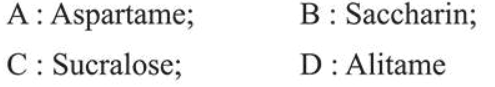2.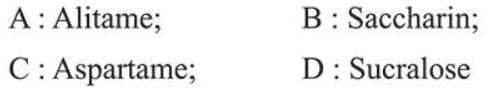3.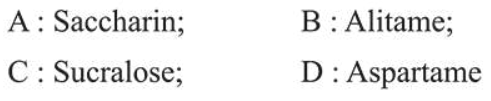4.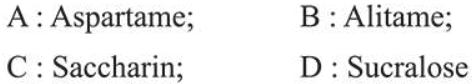Correct Option: 1

Solution: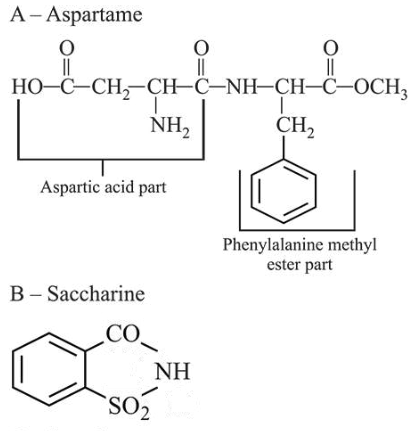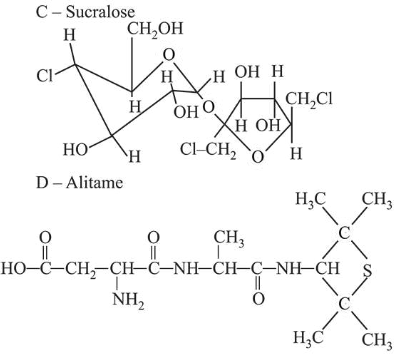(I) A and D give positive test with ninhydrin because both have free carboxylic and amine groups.

(II) $\mathrm{C}$ form precipitate with $\mathrm{Ag} \mathrm{NO}_{3}$ in the lassaigne extract of the sugar because it has chlorine atoms.

(III) B and D give positive test with sodium nitroprusside because both have sulphur atoms.